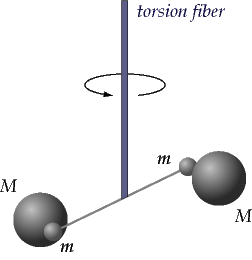# Gravitation - Online Test

Q1. The force of attraction due to a hollow spherical shell of uniform density, on a point mass situated inside it, is
Explaination / Solution:

The gravitational force acting by a spherically symmetric shell upon a point mass inside it, is the vector sum of gravitational forces acted by each part of the shell, and this vector sum is equal to zero. That is, a mass m within a spherically symmetric shell of mass M will feel no net force

Q2. In Cavendish’s experiment,
Explaination / Solution:Cavendish's apparatus for experimentally determining the value of G involved a light, rigid rod about 2-feet long. Two small lead spheres were attached to the ends of the rod and the rod was suspended by a thin wire. When the rod becomes twisted, the torsion of the wire begins to exert a torsional force that is proportional to the angle of rotation of the rod. The more twist of the wire, the more the system pushes backwards to restore itself towards the original position.

Q3. The value of the gravitational constant G is
Explaination / Solution:

The value of G was experimentally determined by Lord Henry Cavendish using a torsion balance.Cavendish brought two large lead spheres near the smaller spheres attached to the rod. Since all masses attract, the large spheres exerted a gravitational force upon the smaller spheres and twisted the rod a measurable amount. Once the torsional force balanced the gravitational force, the rod and spheres came to rest and Cavendish was able to determine the gravitational force of attraction between the masses.Cavendish expressed his result in terms of the density of the Earth.

After converting to SI units, Cavendish's value for the Earth's density, 5.448 g cm−3, gives

G = 6.74×10−11 m3 kg–1 s−2

Today, the currently accepted value is 6.67259 x 10-11 N m2/kg2.

Q4. The acceleration due to gravity at a height h in terms of mass of earth  and radius of the earth  and gravitational constant G is
Explaination / Solution:

We know by Newton's law of gravitation, the force on the body of mass m, situated at height h to the surface of the earth of mass Mis given by:

-(1)

we also know F=mass X acceleration( here acceleration is acceleration due to gravity)

$⇒$ F=mg  -(2)

Equating (i) & (ii), we get

Q5. The acceleration due to gravity at a depth d in terms of g the acceleration due to gravity at radius of the earth   is
Explaination / Solution:

Acceleration due to gravity at the surface of the earth of radius RE

-(1)

Acceleration due to gravity at depth d from the surface of the earth

-(2)

From (1) & (2), we get

Q6. Escape speed from the earth is the
Explaination / Solution:

The escape velocity is the minimum velocity required to leave a planet or moon. For a rocket or other object to leave a planet, it must overcome the pull of gravity.

Vescape=11184 m/sec approximate to 11.2 km/sec

Q7. For earth satellites, the centripetal force is provided by
Explaination / Solution:

Gravity supplies the necessary centripetal force to hold a satellite in orbit about the earth. Setting the gravity force from the universal law of gravity equal to the required centripetal force yields the equation of  motion of satellites in the circular orbit

i.e

Q8. The total energy of a circularly orbiting satellite is
Explaination / Solution:

When a satellite revolves around a planet in its orbit, it possesses both potential energy (due to its position against the gravitational pull of earth) and kinetic energy (due to orbital motion).

Hence we can say that total energy of circularly orbiting satellite is negative.

Q9. For a circularly orbiting satellite the potential energy
Explaination / Solution:

In case of circularly orbiting satellite,

Comparing both, we can say that the potential energy is negative and has a magnitude twice that of the positive kinetic energy.

Q10. For Geostationary Satellites
Explaination / Solution:

If there is a satellite in the equatorial plane rotating in the direction of earth’s rotation from west to east, then for an observer, on the earth, angular velocity of satellite will be

The time interval between the two consecutive appearances overhead will be,

If , i.e. satellite will appear stationary relative to earth. Such satellites are called geostationary satellites and will have a period of rotation as the period of rotation of earth i.e  24 Hrs.